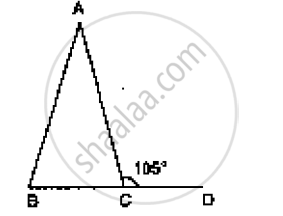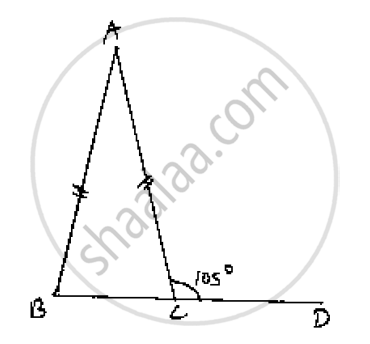# In Fig. 10.24, Ab = Ac and ∠Acd =105°, Find ∠Bac. - Mathematics

In Figure AB = AC and ∠ACD =105°, find ∠BAC.#### SolutionConsider the given figure
We have,
AB = AC and ∠ACD =105^@

Since,

∠BCD = 180° = Straight angle

∠BCA + ∠ACD = 180°

∠BCA +105° = 180°

∠BCA = 180° -105° ⇒ ∠BCA = 75°  .......1

And also,

ΔABC is an isosceles triangle                  [∵AB = AC]

⇒ ∠ABC = ∠ACB

From (1), we have [Angles opposite to equal sides are equal]

∠ACB = 75° ⇒∠ABC = ∠ACB = 75°

And also,

Sum of interior angles of a triangle = 180°

⇒∠ABC = ∠BCA + ∠CAB = 180°

⇒ 75° + 75° + ÐCAB = 180°

⇒ 150° +∠BAC = 180°⇒ ∠BAC = 180° -150° = 30°

∠BAC=30°

Concept: Properties of a Triangle
Is there an error in this question or solution?

#### APPEARS IN

RD Sharma Mathematics for Class 9
Chapter 12 Congruent Triangles
Exercise 12.1 | Q 7 | Page 15

Share